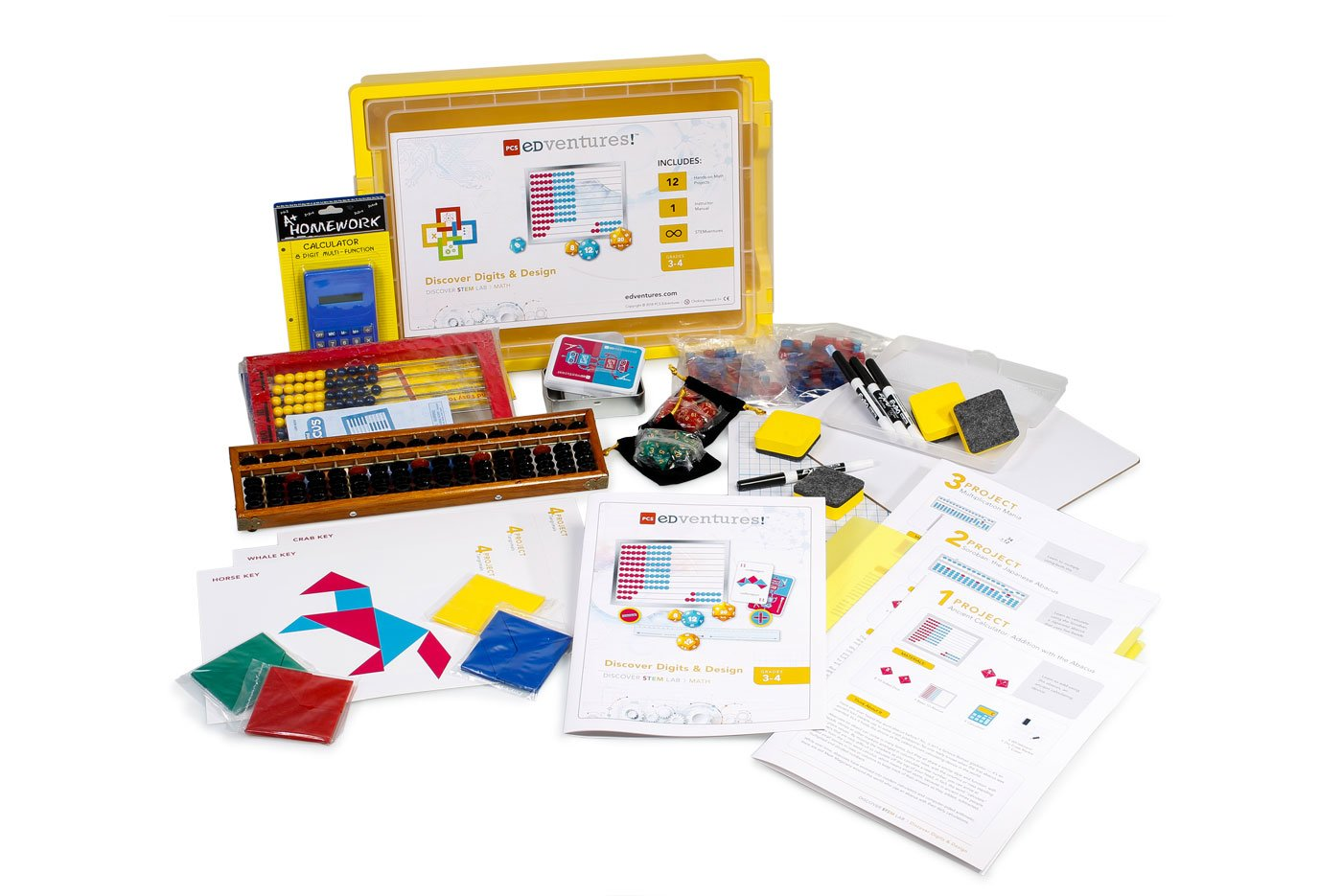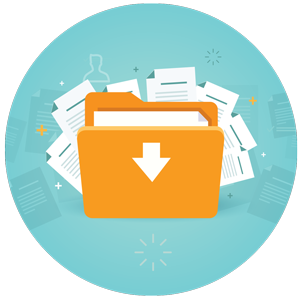# Discover Digits & Design

• 350.00 USD
###### Dive into the Magic of Mathematics!

Model 12 different math activities through hands-on challenges and games. With step-by-step instructions, students work independently with PCS Edventures hands-on math materials, puzzles, tangrams and a student-driven curriculum.

### Highlights:

• Ages: 8-10
• Number of Activities: 12 step-by-step builds and endless open-ended extensions
• Subject Areas: Math Connections
• Student-driven lessons to work independently
• 100% Reusable: Math materials are 100% reusable. Just clean and reuse again and again.

### Student Projects:

1. Learn to add using the abacus, an ancient calculating device.
2. Learn to calculate using the Soroban, a Japanese abacus.
3. Learn to multiply using both the Base-10 Abacus and the Soroban.
4. Piece together animal puzzles using one complete set of tangrams.
5. Construct irregular polygons from a challenging number of tangrams.
6. Complete picture puzzles using two complete sets of tangrams.
7. Learn to add fractions and mixed numbers using a variety of tools.
8. Learn about probability and how it can be expressed as a fraction or ratio.
9. Combine multiplication facts and drawing skills in a fun Array Game.
10. Explore the concept of negative numbers by practicing adding and subtracting on a number line ruler.
11. Learn about positive and negative numbers by playing the classic card game, War, with a twist.
12. Add and subtract both positive and negative integers to hit a target number after exactly ten rolls.

### Complete Program Includes:

• Instructor Guide with Answer Keys: 1
• Student Project Booklets: 12
• Tangram Pieces: 28
• Abacus (base ten): 1
• Abacus (classic soroban): 1
• Calculator: 1
• Dry Erase Markers: 4
• Dry Erasers: 4
• Dry Erase Axis Lap Boards: 4
• Number Line Rulers & Set of 60 Cubes: 2
• PCS Card Deck: 1
• Bags of Polyhedral Dice: 2
• +/- Integer Chip: 1

### Educators & Homeschoolers:## Discover Digits & Design

### PRICING OPTIONS

• 350.00 USD
###### Dive into the Magic of Mathematics!

Model 12 different math activities through hands-on challenges and games. With step-by-step instructions, students work independently with PCS Edventures hands-on math materials, puzzles, tangrams and a student-driven curriculum.

### Highlights:

• Ages: 8-10
• Number of Activities: 12 step-by-step builds and endless open-ended extensions
• Subject Areas: Math Connections
• Student-driven lessons to work independently
• 100% Reusable: Math materials are 100% reusable. Just clean and reuse again and again.

### Student Projects:

1. Learn to add using the abacus, an ancient calculating device.
2. Learn to calculate using the Soroban, a Japanese abacus.
3. Learn to multiply using both the Base-10 Abacus and the Soroban.
4. Piece together animal puzzles using one complete set of tangrams.
5. Construct irregular polygons from a challenging number of tangrams.
6. Complete picture puzzles using two complete sets of tangrams.
7. Learn to add fractions and mixed numbers using a variety of tools.
8. Learn about probability and how it can be expressed as a fraction or ratio.
9. Combine multiplication facts and drawing skills in a fun Array Game.
10. Explore the concept of negative numbers by practicing adding and subtracting on a number line ruler.
11. Learn about positive and negative numbers by playing the classic card game, War, with a twist.
12. Add and subtract both positive and negative integers to hit a target number after exactly ten rolls.

### Complete Program Includes:

• Instructor Guide with Answer Keys: 1
• Student Project Booklets: 12
• Tangram Pieces: 28
• Abacus (base ten): 1
• Abacus (classic soroban): 1
• Calculator: 1
• Dry Erase Markers: 4
• Dry Erasers: 4
• Dry Erase Axis Lap Boards: 4
• Number Line Rulers & Set of 60 Cubes: 2
• PCS Card Deck: 1
• Bags of Polyhedral Dice: 2
• +/- Integer Chip: 1

### Educators & Homeschoolers:## Request Quote

Speak with a STEMbassador to find the best solutions for your environment.## Catalogs

Take this website on the go with a catalog tuned to your STEM needs.## Curriculum Samples

See each course in action with a FREE sample of any product you're interested in.